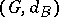# Asymptotic invariant of a group

A property of a finitely-generated groupwhich is a quasi-isometry invariant of the metric space, whereis the word metric associated to a finite generating setof(cf. also Quasi-isometric spaces). This definition does not depend on the choice of the set, since ifis another finite set of generators of, then the metric spacesandare quasi-isometric.
The theory of asymptotic invariants of finitely-generated groups has been recently brought to the foreground by M. Gromov (see, in particular, [a2] and [a3]). As Gromov says in [a3], p. 8, "one believes nowadays that the most essential invariants of an infinite group are asymptotic invariants" . For example, amenability (cf. Invariant average), hyperbolicity (in the sense of Gromov, cf. Hyperbolic group), the fact of being finitely presented (cf. Finitely-presented group), and the number of ends (cf. also Absolute) are all asymptotic invariants of finitely-generated groups. It is presently (1996) unknown whether the Kazhdan-property is an asymptotic invariant. For an excellent survey on these matters, see [a1].